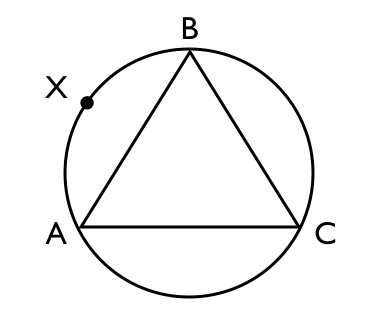Learn the 4 Things You Must Know Before Taking the GMAT!   Download it Now!

# GMAT Geometry: Understanding Pi (w/Applications)

### Happy Pi Day!Okay, okay. We get it. Celebrating this famed mathematical constant is pretty dorky. It’s certainly understandable that Martin Luther King Jr. has a day. It’s even understandable that St. Velentine has a day. But the number 3.14159265359…? Or is it the greek letter π that’s actually being celebrated? No matter, whichever it is, giving it a day of its own is simply irrational!

(See what I did there? Irrational? Because Pi isn’t a rational number? Get it? Hahaha!)

Poor jokes or not, Pi is a pretty extraordinary number when you think about it, and it’s certainly something you absolutely must know something about if you’re going to have any prayer on GMAT quantitative questions involving circles. So, before we go any further, let’s make sure you know what Pi (π) actually is, and how it’s derived. Check out this short video:

Now that you know what Pi is and how it’s determined, let’s be clear about one thing: On the GMAT, all you really need to know about Pi is how to apply it in two important formulas concerning circles. The constant Pi (π) appears in the following two formulas that you absolutely MUST know on the GMAT:

1) The AREA of a Circle = πr² (where r = radius)

So, for example, how would you calculate the area of Circle C in Figure 1 above? Well, the radius (r) = 4, so you simply plug that into the formula and you get that the Area of Circle C = πr² = π(4)² = 16π. Done! Pretty easy. It’s not rocket science.

2) The CIRCUMFERENCE of a Circle = 2πr (where r = radius)

So, for example, how would you calculate the circumference of the circle in Figure 2 above? First, you need to recognize that what’s been given to us is the diameter of the circle, not the radius. But, of course the radius is simply 1/2 the diameter, so we can calculate that the radius of this circle = 3. At that point, it’s simply plug and play. You plug the radius (r) of 3 into the formula and you get that the Circumference of this circle = 2πr = 2π(3) = 6π.

That’s pretty much it. The mathematicians and other high school valedictorians around the world who are truly celebrating Pi today probably know a whole lot more about it than that. But for your purposes to get right answers on the GMAT, which let’s be honest is really all that’s important, knowing the above two formulas inside and out will serve you well and help you get into the business school of your dreams where you can go on to do much more important things…like writing blog articles about the number 3.14159265359…!

With that, try your hand at this sample GMAT geometry question and submit your answer in the “comment” field below. For a full tutorial lesson on GMAT Circles including explanations of how to solve difficult problems like this one, check out our video lesson “GMAT Geometry – Circles.”Question: In the figure above, inscribed triangle ABC is equilateral. If the radius of the circle is r, then the length of arc AXB is

(A) 2πr/3
(B) 4πr/3
(C) 3πr/2
(D) πr² /3
(E) 2πr² /3